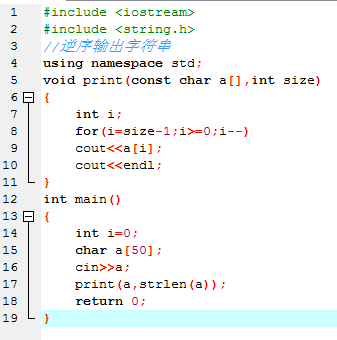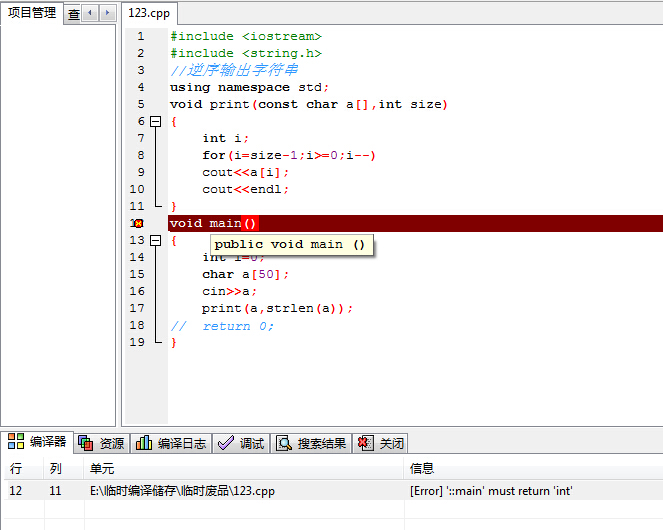C++编程小白求教，逆序输出字符串

#include
#include
#include
#include
//逆序输出字符串
using namespace std;

void print(const char a[],int size){
int i;
for(i=size;i>0;i--)
cout<<a[i-1];
cout<<endl;
}
void main(){

``````int i=0;
char a;
while(getchar()!='\n'){
a[i] = getchar();
i++;
}
print(a,sizeof(a));

while(1);
``````

}

8个回答

`````` #include <iostream>
#include <string.h>
//逆序输出字符串
using namespace std;
void print(const char a[],int size)
{
int i;
for(i=size-1;i>=0;i--)
cout<<a[i];
cout<<endl;
}
int main()
{
int i=0;
char a;
cin>>a;
print(a,strlen(a));
return 0;
}
````````````#include <stdio.h>

void foo()
{
char c = getchar();
if (c == '\n')
return;
foo();
printf("%c", c);
}

int main()
{
foo();
printf("\n");
return 0;
}
``````

for(i=size-1;i>=0;i--)

while循环判定中的getchar()会将第一个字符弹出，所以你无法记录到第一个字符串，而且sizeof(a)，你不能用这个得出有效字符串长度，你只会得出这个数组长度

do{
a[i] = getchar();
i++;
}while(a[i-1]!='\n');

print(a,i-1);

printf( strrev(str) ); //简单明了！！